## Hydrogen Fine Structure

The basic hydrogen problem we have solved has the following Hamiltonian.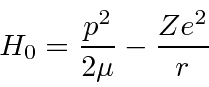To this simple Coulomb problem, we will add several corrections:

1. The relativistic correction to the electron's kinetic energy.
2. The Spin-Orbit correction.
3. The Darwin Term'' correction to s states from Dirac eq.
4. The ((anomalouus) Zeeman) effect of an external magnetic field.

Correction (1) comes from relativity. The electron's velocity in hydrogen is of order. It is not very relativistic but a small correction is in order. By calculating the next order relativistic correction to the kinetic energy we find the additional term in the Hamiltonian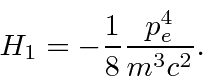Our energy eigenstates are not eigenfunctions of this operator so we will have to treat it as a perturbation.

We can estimate the size of this correction compared to the Hydrogen binding energy by taking the ratio to the Hydrogen kinetic energy. (Remember that, in the hydrogen ground state,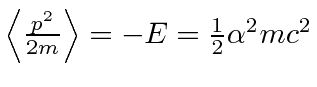.)Like all the fine structure corrections, this is down by a factor of order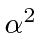from the Hydrogen binding energy.

The second term, due to Spin-Orbit interactions, is harder to derive correctly. We understand the basis for this term. The magnetic moment from the electron's spin interacts with the B field produced by the current seen in the electron's rest frame from the circulating proton.We can derive B from a Lorentz transformation of the E field of a static proton (We must also add in the Thomas Precession which we will not try to understand here).This will be of the same order as the relativistic correction.

Now we compute the relativity correction in first order perturbation theory .The result depends onand, but not onor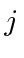. This means that we could use either theor theto calculate the effect of. We will need to use theto add in the spin-orbit.

The first order perturbation energy shift from the spin orbit correction is calculated for the states of definite.Actually, theterm should give 0 for! In the above calculation there is anfactor which makes the result forundefined. There is an additional Dirac Equation contribution called the Darwin term'' which is important forand surprisingly makes the above calculation right, even for!

We will now add these three fine structure corrections together for states of definite. We start with a formula which has slightly different forms for.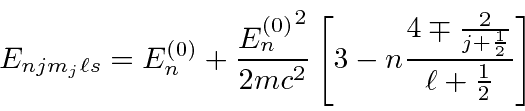We can writeas, so thatand we get a nice cancellation giving us a simple formula.This is independent ofso the states of different total angular momentum split in energy but there is still a good deal of degeneracy.We have calculated the fine structure effects in Hydrogen. There are, of course, other, smaller corrections to the energies. A correction from field theory, the Lamb Shift, causes states of differentto shift apart slightly. Nevertheless, the states of definite total angular momentum are the energy eigenstates until we somehow break spherical symmetry.

Jim Branson 2013-04-22